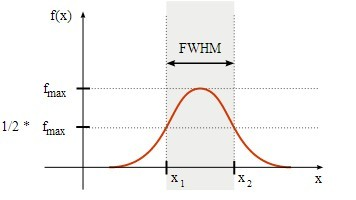English购物车网站地图

## Hz和nm的换算关系，稳频激光器1Hz是多少nm1c=v*λ

2）△v=c/(λ*λ) *△λ

3）△x=c/v

v=c/(λ*λ) *△λ

1Hz=3×108m/s÷（1530nm×1530nm）×△λ

v=c/(λ*λ) *△λ

1Hz=3×108m/s ÷（1560nm×1560nm）×△λ

v=c/(λ*λ) *△λ

1Hz=3×108m/s ÷（632nm×632nm）×△λ